Solving for a Variable Another application of algebra is to solve an equation for a given variable. Example: Using the formula given in Figure 1-12, find the total capacitance (CT) of the series circuit containing three capacitors withTherefore, CT = 1/96.66 = .01034 microfarad. The microfarad (10-6 farad) is a unit of measurement of capacitance. This will be discussed in greater length beginning on page 10-51 in chapter 10, Electricity. Use of Parentheses In algebraic equations, parentheses are used to group numbers or symbols together. The use of parentheses helps us to identify the order in which we should apply mathematical operations. The operations inside the parentheses are always performed first in algebraic equations. Example: Solve the algebraic equation N = (4 + 3)2. First, perform the operation inside the parentheses. That is, 4 + 3 = 7. Then complete the exponent calculation N = (7)2 = 7 x 7 = 49. When using more complex equations, which may combine several terms and use multiple operations, grouping the terms together helps organize the equation. Parentheses, ( ), are most commonly used in grouping, but you may also see brackets, [ ]. When a term or expression is inside one of these grouping symbols, it means that any operation indicated to be done on the group is done to the entire term or expression.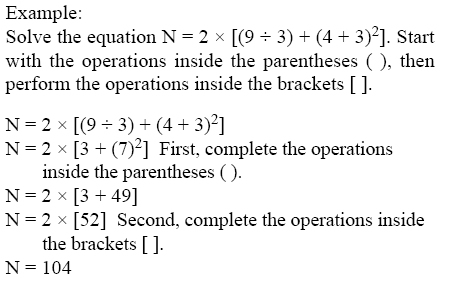Order of Operation In algebra, rules have been set for the order in which operations are evaluated. These same universally accepted rules are also used when programming algebraic equations in calculators. When solving the following equation, the order of operation is given below: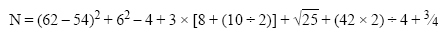Parentheses. First, do everything in parentheses, ( ). Starting from the innermost parentheses. If the expression has a set of brackets, [ ], treat these exactly like parentheses. If you are working with a fraction, treat the top as if it were in parentheses and the denominator as if it were in parentheses, even if there are none shown. From the equation above, completing the calculation in parentheses gives the following: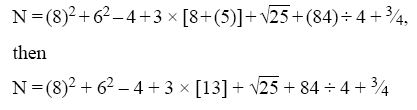Exponents. Next, clear any exponents. Treat any roots (square roots, cube roots, and so forth) as exponents. Completing the exponents and roots in the equation gives the following: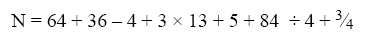Multiplication and Division. Evaluate all of the multiplications and divisions from left to right. Multiply and divide from left to right in one step. A common error is to use two steps for this (that is, to clear all of the multiplication signs and then clear all of the division signs), but this is not the correct method. Treat fractions as division. Completing the multiplication and division in the equation gives the following: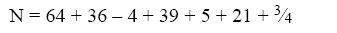Addition and Subtraction. Evaluate the additions and subtractions from left to right. Like above, addition and subtraction are computed left to right in one step. Completing the addition and subtraction in the equation gives the following: X = 161 3⁄4
 ©AvStop Online Magazine                                                                                                                                                      Contact Us              Return To Books